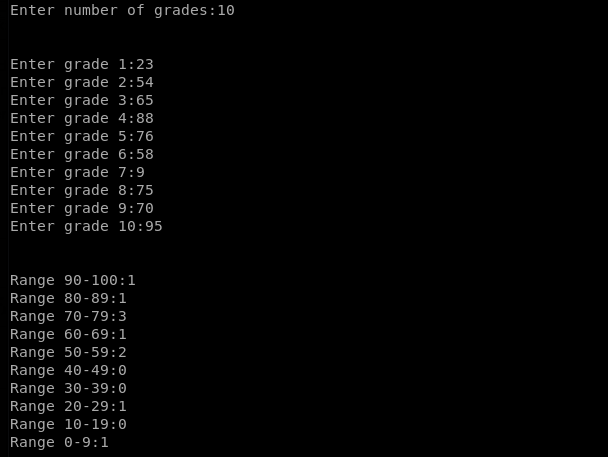# Program to Count the Grades that lie between a certain Range

Program to Count the Grades that lie between a certain Range

Below is the Python code whose output matches the desired output. The program:-

– validates input of the number of grades so that only a number greater than 0 and less than 100 is entered;
counts the number of grades that lie between a certain range; and
prints the count of these numbers in respective ranges.

```#!/usr/bin/python3

print("\n")

n = 1
x = 0
a = b = c = d = e = f = g = h = i = j = 0
n+=1

if int(x) >=90 and int(x)<=100:
j += 1
elif int(x) >=80 and int(x)<90:
i += 1
elif int(x) >=70 and int(x)<80:
h += 1
elif int(x) >=60 and int(x)<70:
g += 1
elif int(x) >=50 and int(x)<60:
f += 1
elif int(x) >=40 and int(x)<50:
e += 1
elif int(x) >=30 and int(x)<40:
d += 1
elif int(x) >=20 and int(x)<30:
c += 1
elif int(x) >=10 and int(x)<20:
b += 1
else:
a += 1

print("\n\nRange 90-100:" + str(j))
print("Range 80-89:" + str(i))
print("Range 70-79:" + str(h))
print("Range 60-69:" + str(g))
print("Range 50-59:" + str(f))
print("Range 40-49:" + str(e))
print("Range 30-39:" + str(d))
print("Range 20-29:" + str(c))
print("Range 10-19:" + str(b))
print("Range 0-9:" + str(a) + "\n")

else:
print("Enter a number greater than 0!")```

Below is the Python code output screenshot;Program to Count the Grades that lie between a certain Range
Python | thetqweb